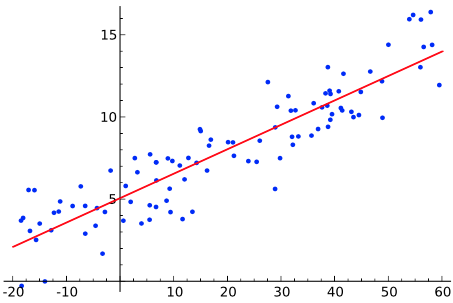# 最小二乘法求解直线方程系数

## 引言## 直线方程最优拟合

1. 斜率为k$k$
2. y$y$轴截距为b$b$

fk=ni=1[(yikxib)xi]=0fb=ni=1(yikxib)=0

{ni=1(xiyi)kni=1(x2i)bni=1(xi)=0ni=1(yi)kni=1(xi)nb=0

{Ak+bB=CBk+nb=D

x1x2xn111p1p2pn=y1y2yn

## 总结